Processing ......FreeComputerBooks.com Links to Free Computer, Mathematics, Technical Books all over the World

Principles of Algorithmic Problem Solving
GIS Visualizer - Geographic Data Visualized on 40+ Maps! Click here for details.
• Title: Principles of Algorithmic Problem Solving
• Author(s) Johan Sannemo
• Publisher: KTH Royal Institute of Technology (2018)
• Hardcover/Paperback: N/A
• eBook: PDF (351 pages)
• Language: English
• ISBN-10/ASIN: N/A
• ISBN-13: N/A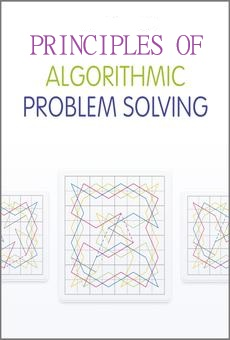Book Description

Algorithmic problem solving is the art of formulating efficient methods that solve problems of a mathematical nature. The purpose of this book is to contribute to the literature of algorithmic problem solving in two ways. First of all, it tries to fill in some holes in existing books. Secondly, it provides another way of learning the basics of algorithmic problem solving by helping the reader build an intuition for problem solving.

The algorithmic approach to solving problems in computer technology is an essential tool. This unique book teaches the fundamental principles of using algorithms to solve problems. It presents a readable, entertaining, and energetic book that will motivate and challenge students to open their minds to the algorithmic nature of problem solving.

• Provides a novel approach to the mathematics of problem solving focusing on the algorithmic nature of problem solving
• Uses popular and entertaining puzzles to teach you different aspects of using algorithms to solve mathematical and computing challenges
• Features a theory section that supports each of the puzzles presented throughout the book
• Assumes only an elementary understanding of mathematics
• N/A
Reviews, Ratings, and Recommendations: Related Book Categories: Read and Download Links:Similar Books:
•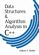Data Structures and Algorithm Analysis in C++, 3rd Edition

A comprehensive treatment focusing on the creation of efficient data structures and algorithms, using C++. This text explains how to select or design the data structure best suited to specific problems.

•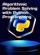Algorithmic Problem Solving with Python (John B. Schneider)

This book uses Python to introduce folks to programming and algorithmic thinking. It is sharply focused on classical algorithms, but it also gives a solid understanding of fundamental algorithmic problem-solving techniques.

•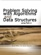Problem Solving with Algorithms and Data Structures Using Python

This is a textbook about computer science. It is also about Python. However, there is much more. The tools and techniques that you learn here will be applied over and over as you continue your study of computer science.

•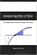Annotated Algorithms in Python: with Applications

This book covers Analysis and Design of Algorithms, Scientific Computing, Monte Carlo Simulations, and Parallel Algorithms. It teaches the core knowledge required by any scientist interested in numerical algorithms and computational finance.

•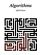Lecture Notes for the Algorithms (Jeff Erickson)

This lecture notes uniquely combines rigor and comprehensiveness. It covers a broad range of algorithms in depth, yet makes their design and analysis accessible to all levels of readers. Each chapter is relatively self-contained and can be used as a unit of study.

•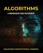Algorithms: Fundamental Techniques (Macneil Shonle, et al)

The goal of the book is to show you how you can methodically apply different techniques to your own algorithms to make them more efficient. While this book mostly highlights general techniques, some well-known algorithms are also looked at in depth.

•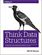Think Data Structures: Algorithms and Information Retrieval

This practical book will help you learn and review some of the most important ideas in software engineering - data structures and algorithms - in a way that's clearer, more concise, and more engaging than other materials. Useful in technical interviews too.

•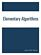Elementary Algorithms (Xinyu Liu)

This book doesn't only focus on an imperative (or procedural) approach, but also includes purely functional algorithms and data structures. It teaches you how to think like a programmer - find the practical efficiency algorithms to solve your problems.

•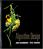Algorithm Design (Jon Kleinberg, et al)

This book introduces algorithms by looking at the real-world problems that motivate them. The book teaches a range of design and analysis techniques for problems that arise in computing applications.

•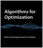Algorithms for Optimization (Mykel J. Kochenderfer, et al.)

A comprehensive introduction to optimization with a focus on practical algorithms for the design of engineering systems. Readers will learn about computational approaches for a range of challenges.

•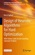Design of Heuristic Algorithms for Hard Optimization

This open access book demonstrates all the steps required to design heuristic algorithms for difficult optimization. The classic problem of the travelling salesman is used as a common thread to illustrate all the techniques discussed.

•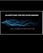Algorithms for Decision Making (Mykel Kochenderfer, et al)

This textbook provides a broad introduction to algorithms for decision making under uncertainty, covering the underlying mathematical problem formulations and the algorithms for solving them.

•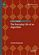The Everyday Life of an Algorithm (Daniel Neyland)

Through investigating the everyday life of the algorithm, the book opens a conversation with existing social science research that tends to focus on the power and opacity of algorithms, via unique access to the algorithm's design, development, testing, etc.

Book Categories
 :All CategoriesTop Free BooksRecent BooksMiscellaneous BooksComputer EngineeringComputer LanguagesComputer ScienceData Science/DatabasesJava and Java EE (J2EE)Linux and UnixMathematicsMicrosoft and .NETMobile ComputingNetworking and CommunicationsSoftware EngineeringSpecial TopicsWeb Programming
Other Categories